# PSAT Math : Fractions

## Example Questions

← Previous 1 3 4 5 6 7 8 9 17 18

### Example Question #1 : Proportion / Ratio / Rate

A cafeteria with 40 tables can sit 600 people. Some tables can sit 10 people and some can sit 20 people. What is the ratio of the number of 10-person tables to the number of 20-person tables?

2:1

1:4

1:1

1:2

4:1

1:1

Explanation:

Let x be the number of 10-person tables, and y be the number of 20-person tables. Since there are 40 tables in the cafeteria, x + y = 40. 10x represents the number of people sitting at 10-person tables, and 20y represents the number of people sitting at 20-person tables. Since the cafeteria can seat 600 people, 10x + 20y = 600. Now we have 2 equations and 2 unknowns, and can solve the system. To do this, multiply the first equation by 10 and subtract it from the second equation. This yields 0x + 10y = 200; solving for y tells us there are 20 tables that seat 20 people. Since x + y = 40, x = 20, so there are 20 tables that seat 10 people. The ratio of x:y is therefore 1:1.

### Example Question #2 : Proportion / Ratio / Rate

The first term in a sequence is m. If every term thereafter is 5 greater than 1/10 of the preceding term, and m≠0, what is the ratio of the second term to the first term?

(m+10)/5

(m+10)/50m

(m+50)/10m

(m+5)/10

(m+50)/10m

Explanation:

The first term is m, so the second term is m/10+5 or (m+50)/10. When we take the ratio of the second term to the first term, we get (((m+50)/10))/m, which is ((m+50)/10)(1/m), or (m+50)/10m.

### Example Question #3 : Proportion / Ratio / Rate

Two cars were traveling 630 miles. Car A traveled an average speed of 70 miles per hour. If car B traveled 90 miles an hour, how many miles had car A traveled when car B arrived at the destination?

140

700

490

630

490

Explanation:

We first divide 630 miles by 90 miles per hour to get the amount of time it took car B to reach the destination, giving us 7 hours. We then multiply 7 hours by car A’s average speed and we get 490 miles.

### Example Question #4 : Proportion / Ratio / Rate

 STUDENT ATHLETES WHO USE STEROIDS MEN WOMEN TOTAL BASKETBALL A B C SOCCER D E F TOTAL G H I

In the table above, each letter represents the number of students in each category. Which of the following must be equal to I?

A+B

G+H

A+D

A+D+G

G+H

Explanation:

Since G is the total number of male athletes that use steroids and H is the total number of female athletes that use steroids, the sum of the two is equal to I, which is the total number of all students using steroids.

### Example Question #1 : Proportion / Ratio / Rate

A particular ball always bounces back to 2/5 of the height of its previous bounce after being dropped. After the first bounce it reaches a height of 175 inches. Approximately how high (in inches) will it reach after its fifth bounce?

4.5

14

11.2

20

4.5

Explanation:

The first bounce reaches a height of 175. The second bounce will equal 175 multiplied by 2/5 or 70. Repeat this process. You will get the data below. 4.48 is rounded to 4.5.

### Example Question #1 : Arithmetic

A lawn can be mowed by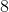people in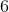hours. If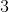people take the day off and do not help mow the grass, how many hours will it take to mow the lawn?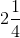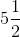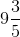Explanation:

The number of hours required to mow the lawn remains constant and can be found by taking the originalworkers times thehours they worked, totaling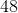hours. We then split the total required hours between the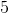works that remain, and each of them have to work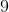and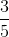hours: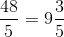.

### Example Question #6 : Proportion / Ratio / Rate

The flow of water through a certain pipe is 20 cubic meters per minute.  How many minutes would it take for 4 of such pipes to fill 2 tanks, if each tank is a cube with a side length of 20 m?

200

40

50

100

20

200

Explanation:

The flow of water through one pipe is 20 m/ minute.

Thus, the flow of water through 4 pipes is 80 m3 / minute.

Since each tank is a cube with a side length of 20m, the volume of each tank is:

Volume of one tank = (20 m)3 = 8000 m3.

The total volume of two tanks is 2 * 8000 m= 16,000 m3

Therefore, the total minutes for 4 pipes to fill 2 tanks is:

16,000 m3/(80 m3/min) = 200 minutes

80 m3/min

### Example Question #1 : Proportion / Ratio / Rate

You are planning a party.  The maximum number of people the reception hall can hold is 1 person for every 5 square feet of space.  If the hall is 60 feet wide and 50 feet long, how many people can you invite?

3000

2500

500

1500

600

600

Explanation:

Total area of hall = 60ft * 50ft = 3000 ft2

At 1 person per 5 square feet, 3000 ft2 / 5 ft2 per person  = 600 people

### Example Question #1 : Proportion / Ratio / Rate

A gallon contains 8 pints.  Each pint contains 2 cups.  How many cups are in a 10-gallon jug of water?

20

220

26

80

160

160

Explanation:

Find the number of cups in a gallon, then calculate cups in 10 gallons.

If 8 pints = 1 gallon, then 16 cups = 1 gallon

16 cups * 10 gallons = 160 cups

### Example Question #1 : Proportion / Ratio / Rate

A mile is 5280 feet

Susan is able to walk a fast pace of 4 miles per hour.  How many feet will she walk in 40 minutes?

8/3

15000

14080

15840

3

14080

Explanation:

Calculate the number of feet walked in an hour.  Then calculate what fraction of an hour 40 minutes is.

5280 * 4 = 21120 feet walked in an hour

60 minutes in an hour, so 40 minutes = 2/3 hour (40/60)

21120(2/3) = 14080 feet walked in 40 minutes

← Previous 1 3 4 5 6 7 8 9 17 18

### All PSAT Math Resources